# CONFUSION MATRIX

The confusion matrix is a matrix used to determine the performance of the classification models for a given set of test data. It can only be determined if the true values for test data are known. The matrix itself can be easily understood, but the related terminologies may be confusing. Since it shows the errors in the model performance in the form of a matrix, hence also known as an error matrix. Some features of Confusion matrix are given below:

• For the 2 prediction classes of classifiers, the matrix is of 2*2 table, for 3 classes, it is 3*3 table, and so on.
• The matrix is divided into two dimensions, that are predicted values and actual values along with the total number of predictions.
• Predicted values are those values, which are predicted by the model, and actual values are the true values for the given observations.
• It looks like the below table:

he above table has the following cases:

• True Negative: Model has given prediction No, and the real or actual value was also No.
• True Positive: The model has predicted yes, and the actual value was also true.
• False Positive: The model has predicted Yes, but the actual value was No. It is also called a Type-I error.
• False Negative: The model has predicted no, but the actual value was Yes, it is also called as Type-II error.

# Calculations using Confusion Matrix:

We can perform various calculations for the model, such as the model’s accuracy, using this matrix. These calculations are given below:

• Classification Accuracy: It is one of the important parameters to determine the accuracy of the classification problems. It defines how often the model predicts the correct output. It can be calculated as the ratio of the number of correct predictions made by the classifier to all number of predictions made by the classifiers. The formula is given below:
• Misclassification rate: It is also termed as Error rate, and it defines how often the model gives the wrong predictions. The value of error rate can be calculated as the number of incorrect predictions to all number of the predictions made by the classifier. The formula is given below:
• Precision: It can be defined as the number of correct outputs provided by the model or out of all positive classes that have predicted correctly by the model, how many of them were actually true. It can be calculated using the below formula:
• Recall: It is defined as the out of total positive classes, how our model predicted correctly. The recall must be as high as possible.
• F-measure: If two models have low precision and high recall or vice versa, it is difficult to compare these models. So, for this purpose, we can use F-score. This score helps us to evaluate the recall and precision at the same time. The F-score is maximum if the recall is equal to the precision. It can be calculated using the below formula:

# CYBER ATTACK

Cyber-attacks have become one of the biggest problems of the world. They cause serious financial damages to countries and people every day. The increase in cyber-attacks also brings along cyber-crime. The key factors in the fight against crime and criminals are identifying the perpetrators of cyber-crime and understanding the methods of attack. Detecting and avoiding cyber-attacks are difficult tasks. However, researchers have recently been solving these problems by developing security models and making predictions through artificial intelligence methods. A high number of methods of crime prediction are available in the literature. On the other hand, they suffer from a deficiency in predicting cyber-crime and cyber-attack methods. This problem can be tackled by identifying an attack and the perpetrator of such attack, using actual data. The data include the type of crime, gender of perpetrator, damage and methods of attack. The data can be acquired from the applications of the persons who were exposed to cyber-attacks to the forensic units.

They have discussed how various Machine Learning Algorithms are used to tackle this attacks there they have also discussed about Confusion Matrix

The importance of the fight against such cyber-attacks, cyber-crimes and cyber security is highlighted in various studies. Cyber security is the protection of physical-digital data, networks, and technological systems from cyber-attacks, unauthorized accesses, disruptions, modifications, destructions and damages through various processes, applications and applied technologies (Fischer, 2009). Cyber-attacks such as distributed denial of service attacks by sending malicious packets (Kaur Chahal, Bhandari & Behal, 2019), phishing attacks to banking and shopping sites that deceive the user (Sahingoz et al., 2019) have increased significantly. In addition, attackers have been using malicious attack software (virus, worms, trojans, spyware and ransomware) that is installed into the user’s computer without any consent of the user (Biju, Gopal & Prakash, 2019) increasingly. Again, the most common of these attacks and one of the attacks that are most difficult to be prevented is the social engineering attacks.

Accuracy (Acc) score is a method used to evaluate the performance of the model made by comparing the predictions made after running the algorithm with the test data. A value between 0 and 1 is produced according to the ratio of the entire predicted value for a prediction to match with the real values. To determine the accuracy of the forecast:

• TP = Prediction is positive(normal) and actual is positive(normal).
• FP = Prediction is positive(normal) and actual is negative(abnormal).
• FN = Prediction is negative(abnormal) and actual is positive(normal).
• TN = Prediction is negative(abnormal) and actual is negative(abnormal)

The other evaluation metrics for the proposed model are precision, recall and F1-score. Precision (P) is the rate of correctly classified positive instances to the total number of positive instances. Recall (R) shows how successfully positive instances are predicted. F1-Score (F1) is the weighted average of the Precision and Recall values.

Acc=TP+TNTP+TN+FP+FN

P=TPTP+FP

R=TPTP+FN

F1=2TP2TP+FN+FP

In this section, results obtained by use of SVM (Linear), RF, Logistic Regression, XGBoost, SVM (Kernel), DT, KNN, NB algorithms are presented. We can evaluate the Pearson correlation coefficient between these data as shown in Fig. This correlation matrix shows that there are substantial correlations between practically all pairs of variables.

This suggests a method that predicts and detects cyber-attacks by using both machine-learning algorithms and the data from previous cyber-crime cases. In the model, the characteristics of the people who may be attacked and which methods of attack they may be exposed to are predicted.

THANK YOU !!!

--

--

--

Love podcasts or audiobooks? Learn on the go with our new app.

## How to teach a machine to identify the numbers in the image using KERAS library## How To Deploy A Neural Network From Beirut## Topic Modeling in Power BI using PyCaret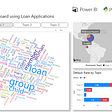## Machine Learning DevOps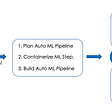## A tutorial on digit recognition with Random Forest Classifier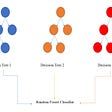## Journey towards building trashbot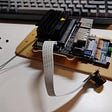## 10 Types of Cross-Validation in Machine Learning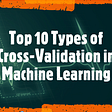## Analyzing the massive appeal of Squid Game## Sentiment Analyis Using AWS## “Summary of 2021 “ from Storange Cloud